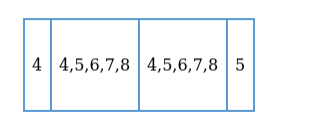# How many numbers divisible by 5 and lying between 4000 and 5000 can be

Question:

How many numbers divisible by 5 and lying between 4000 and 5000 can be formed from the digits 4, 5, 6, 7, 8 if repetition of digits is allowed?

Solution:

Given: We have 5 digits, i.e. 4,5,6,7,8

To Find: Number of numbers divisible by 5

Condition: (i) Number should be between 4000 and 5000

(ii) Repetition of digits is allowed

Here as the number is lying between 4000 and 5000 , we can conclude that the number is of 4 -digits and the number must be starting with 4 .

Now, for a number to be divisible by 5 must ends with 5

Let us represent the 4-digit numberTherefore,

The first place is occupied by $4=1$ way

The fourth (last) place is occupied by $5=1$ way

The second place can be filled by 5 numbers $=5$ ways

The third place can be filled by 5 numbers $=5$ ways

Total numbers formed $=1 \times 5 \times 5 \times 1=25$

There are 25 numbers which are divisible by 5 and lying between 4000 and 5000 and can be formed from the digits $4,5,6,7,8$ with repetition of digits.